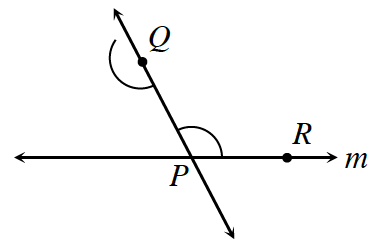### Home > GC > Chapter 9 > Lesson 9.2.3 > Problem9-84

9-84.Multiple Choice: Jamila has started to construct a line parallel to line $m$ through point $Q$ at right. Which of the possible strategies below make the most sense to help her find the line parallel to $m$ through point $Q$?

1. Measure $∠QPR$ with a protractor.

2. Use the compass to measure the arc centered at $P$, then place the point of the compass where the arc centered at $Q$ meets $\overline{QP}$, and mark that measure off on the arc.

3. Construct $\overline{QR}$.

4. Measure $PR$ with a ruler.

Drawing a line parallel to $m$ through point $Q$ would create a corresponding angle congruent to $∠QPR$.

(b)